# The Post Correspondence Programming Language: Domino-oriented Programming

## A Simple Puzzle

Suppose you are given infinitely many of each of the following dominos: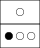1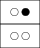2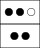3

Can you find a way to arrange some of the dominos so that the sequence of dots on top row matches the bottom row? Here is one possible solution:3231

This list of dominos is called a match since reading off the top row we get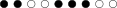which is the same as reading off the bottom row.

Determining whether a collection of dominos has a match is known as the Post Correspondence Problem, or PCP for short. Despite its simplicity, we can use PCP to compute mathematical functions by cleverly picking the dominos that are used.

## Computation via Dominos

To demonstrate its potential for computation, we show how PCP can be used to calculate the least common multiple of two integers.

Theorem: To compute the least common multiple of integers a and b, solve the Post Correspondence Problem for the dominos: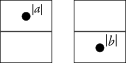The number of dots in the shortest match is LCM(a, b).

The proof of this theorem is left as an exercise, but here is an example shortest match for a = 4 and b = 6: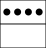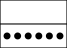The number of dots in the top and bottom rows is LCM(4, 6) = 12.

What else, besides LCM, can we compute using PCP? The answer is surprising: any computation that is doable on a Turing machine is doable using PCP. This implies that PCP, like Turing machines, can be used for general-purpose programming.

To emphasize PCP as programming language, we will refer to it as the Post Correspondence Programming Language, or PCPL for short. This allows us to elegantly formalize PCP's computational power:

Theorem: PCPL is Turing-complete.

A proof of this theorem can be found in Michael Sipser's textbook Introduction to the Theory of Computation, second edition, section 5.2. This proof describes a series of steps to compile any Turing machine into an equivalent PCP instance (PCPL program). We've followed these steps to construct the PCPL program in the following example.

## PCPL Example

Let M be a Turing machine that implements unary addition. Here is the PCPL program compiled from M:

#
#x\$IN#
x+
1y
x1
1x
y1
1y
z+
_a
z1
_a
_y_
z__
1y_
z1_
+y_
z+_
_
_
1
1
+
+
#
#
#
_#
_a
a
a_
a
1a
a
a1
a
+a
a
a+
a
a##
#

These dominos differ slightly from our previous examples. For clarity, we allow arbitrary symbols on dominos in PCPL, rather than limiting ourselves to dots. We also force the PCP match to start with the first domino, with the string `\$IN` replaced by the input to the program.

The PCPL program is constructed so that a match exists if and only if M halts in an accept state for the given input. Finding a match actually forces a simulation of M. This is what gives PCPL its computational power. To see how, let's compute `1+11` by finding a match:

#
#x1+11#
x1
1x
+
+
1
1
1
1
#
#
1
1
x+
1y
1
1
1
1
#
#
1
1
1
1
y1
1y
1
1
#
#
1
1
1
1
1
1
y1
1y
#
_#
1
1
1
1
1
1
1y_
z1_
#
#
1
1
1
1
1
1
z1
_a
_
_
#
#
1
1
1
1
1
1
_
_
a_
a
#
#
1
1
1
1
1
1
_a
a
#
#
1
1
1
1
1a
a
#
#
1
1
1a
a
#
#
1a
a
#
#
a##
#

The string on the top and bottom row is:

`#x1+11#1x+11#11y11#111y1#1111y_#111z1_#111_a_#111_a#111a#11a#1a#a##`

This string is an accepting computation history for M. The `#` symbol separates configurations of M:

1. x1+11
2. 1x+11
3. 11y11
4. 111y1
5. 1111y_
6. 111z1_
7. 111_a_
8. 111_a
9. 111a
10. 11a
11. 1a
12. a

Strings 8 through 12 represent uninteresting clean-up steps, so we will ignore them. Each of the remaining strings is a configuration of M that captures the machine's current state (either x, y, z, or a), the position of the machine head (to the left of the state letter), and the contents of the machine's tape (the rest of the string). Strings 1 through 7 represent the sequence of steps that M would have taken, if it were run on the input `1+11` directly. In this case, the machine halts in state a, an accepting state, with the result `111`.

## PCPL Implementation

The Haskell PCPL package implements the Turing machine to PCPL compiler described in Sipser's proof. The package also provides a generic PCP solver that is used to execute PCPL programs. Once you've installed PCPL (instructions below) and read the API documentation, the easiest way to interact with the package is via GHCI:

``λ> import Language.PCPL``

This module exports the unary adder example from above:

``````λ> :type unaryAdder

We can compile the Turing machine into a PCPL program as follows:

``````λ> :type compileTM
compileTM :: TuringMachine -> Program

λ> let pgm = compileTM unaryAdder
λ> pgm
Program [
{# | #x\$IN#}, {x+ | 1y}, {x1 | 1x}, {y1 | 1y}, {z+ | _a},
{z1 | _a}, {_y_ | z__}, {1y_ | z1_}, {+y_ | z+_}, {_ | _},
{1 | 1}, {+ | +}, {# | #}, {# | _#}, {_a | a}, {a_ | a},
{1a | a}, {a1 | a}, {+a | a}, {a+ | a}, {a## | #}
]``````

Now we can use the `runProgram` function to execute the program. Behind the scenes, this function does a naive breadth-first search to find a match.

``````λ> :type runProgram
runProgram :: Program -> Input -> [Domino]

λ> let input = syms "11+111"
λ> input
[Symbol "1",Symbol "1",Symbol "+",Symbol "1",Symbol "1",Symbol "1"]

λ> let match = runProgram pgm input
λ> length match
94
λ> topString match
"#x11+111#1x1+111#11x+111#111y111#1111y11#11111y1#111111y_#11111z1_
#11111_a_#11111_a#11111a#1111a#111a#11a#1a#a##"``````

The `Language.PCPL` module also exports a Turing machine that only accepts strings of balanced parentheses. The input to this Turing machine must start with a `\$` symbol due to a peculiarity that is described in the API docs.

``````λ> topString \$ runProgram (compileTM parensMatcher) (syms "\$()")
"#S\$()#\$0()#\$(0)#\$1(A#\$A0A#\$AA0_#\$A2A_#\$2AA_#2\$AA_#\$YAA_#\$YA_#\$Y_#\$Y#Y##"``````

If we run the program on a string with unbalanced parentheses, the program never terminates since a match does not exist.

``````λ> runProgram (compileTM parensMatcher) (syms "\$(")
...``````

## Building and Installing PCPL

The PCPL Haskell package depends on the utm package. To install everything at once, run the following commands:

``````\$ git clone https://github.com/davidlazar/utm
\$ git clone https://github.com/davidlazar/PCPL
\$ cabal install utm/ PCPL/``````